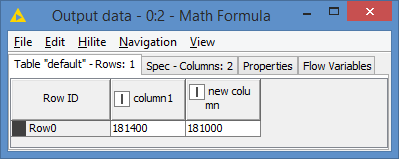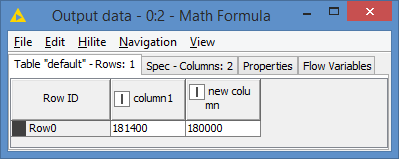# How do I round a whole number to the nearest 1,000?

For example in excel I can use MROUND to change the figure 181,400 to 180,000 by using the MROUND function.

How do I do similar in KNIME?

Thank you.

Hi @JaySmith123 , in your title, you said you want to round to the nearest 1,000, but in your example, you are actually rounding to the nearest 10,000.

181,400 would be rounded to 181,000 if it was to the nearest 1,000, but to 180,000 to the nearest 10,000.

Just take 1,400 as an example. It would be rounded to 1,000 to the nearest 1,000, and 0 to the nearest 10,000.

There is a round() function in Knime that rounds decimal to integer, but I’m not sure if there is a function for rounding to the nearest 1,000 or 10,000. You can always implement the logic manually.

Let’s assume your nearest value is defined by x (so x is either 1,000 or 10,000 depending on which one you meant). Just do a division by x, round the result, and multiply by x. So the formula would be:
`round(your_value/x) * x`

For example, if I round to the nearest 1,000:
`round(\$column1\$/1000) * 1000`

Results:And rounded to the nearest 10,000:
`round(\$column1\$/10000) * 10000`

Results:9 Likes

Hi, thanks so much it worked and sorry for the mistake I did mean 10,000 rounding so I just amended your solution a bit to fit my needs.

Really appreciate it

1 Like

No problem @JaySmith123 , happy to helpThis topic was automatically closed 7 days after the last reply. New replies are no longer allowed.